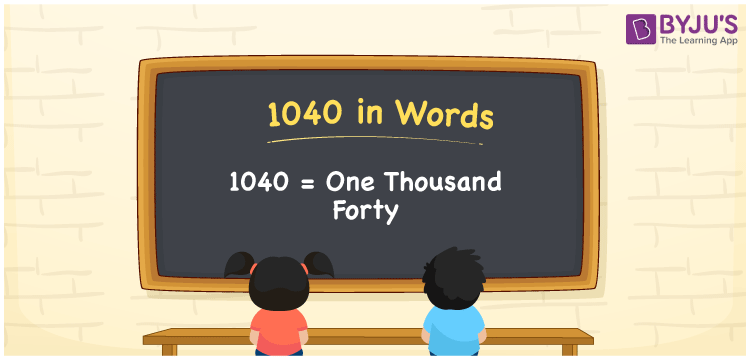# 1040 in Words

1040 in words is written as One thousand forty. In both the International System of Numerals and the Indian System of Numerals, 1040 is written as One thousand forty. The number 1040 is a Cardinal Number as it represents some quantity. For example, “that item costs 1040 rupees”.

 1040 in Words One thousand forty One thousand forty in numerical form 1040

## 1040 in English Words

1040 in English words is read as “One thousand forty”.## How to Write 1040 in Words?

To write 1040 in words, we shall use the place value chart. In the place value chart, put 1 in the thousands, 0 in the hundreds, 4 in the tens, and 0 in the ones, respectively. Let us make a place value chart to write the number 1040 in words.

 Thousands Hundreds Tens Ones 1 0 4 0

Thus, we can write the expanded form as

1 × Thousand + 0 × Hundred + 4 × Ten + 0 × One

= 1 × 1000 + 0 × 100 + 4 × 10 + 0 × 1

= 1000 + 0 + 40 + 0

= 1040

= One thousand forty.

1040 is a natural number which is the successor of 1039 and the predecessor of 1041.

1040 in words – One thousand forty

• Is 1040 an odd number? – No
• Is 1040 an even number? – Yes
• Is 1040 a perfect square number? – No
• Is 1040 a perfect cube number? – No
• Is 1040 a prime number? – No
• Is 1040 a composite number? – Yes

## Frequently Asked Questions on 1040 in Words

Q1

### How to write 1040 in words?

1040 in words is written as One thousand forty.
Q2

### How to write 1040 in words in the International and Indian System of Numerals?

In both, the system of numerals, 1040 in words, is written as One thousand forty.
Q3

### How to write 1040 in a place value chart?

In the place value chart, write 1 in the thousands, 0 in the hundreds, 4 in the tens, and 0 in the ones, respectively.
Test your Knowledge on 1040 in Words